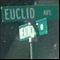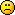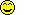Discussion about math, puzzles, games and fun.   Useful symbols: ÷ × ½ √ ∞ ≠ ≤ ≥ ≈ ⇒ ± ∈ Δ θ ∴ ∑ ∫  π  -¹ ² ³ °

You are not logged in.

#1 2007-10-17 11:08:24

Xerxes
Guest

Help dividing polynomial by trinomial

The problem is

So far, the work I have is:

I'm stuck here, as I don't know what to do with the 2 to get

Help!

#2 2007-10-17 11:31:35

Ricky
ModeratorRegistered: 2005-12-04
Posts: 3,791

Re: Help dividing polynomial by trinomial

What you want to do is polynomial division.  Basically the overall view is that you teach each component (ax^n) as a different digit and the rest follows like normal division.  Follow the steps in this link, and feel free to ask questions if you don't understand part of it.

http://www.sosmath.com/algebra/factor/fac01/fac01.html

"In the real world, this would be a problem.  But in mathematics, we can just define a place where this problem doesn't exist.  So we'll go ahead and do that now..."

Offline

#3 2007-10-17 11:44:12

Xerxes
Guest

Re: Help dividing polynomial by trinomial

Yeah, I can divide by a binomial fine, but can't do the trinomial. In this specific example, nothing times 2 gives you 3. Not a whole number, anyway. That's where I am stuck. I have the answer, but don't see where it's coming from.

Can anyone show me the work for the second number in the answer?

#4 2007-10-17 12:11:39

JaneFairfax
MemberRegistered: 2007-02-23
Posts: 6,868

Offline

#5 2007-10-17 13:10:28

Xerxes
Guest

Re: Help dividing polynomial by trinomial

Yes, I know the answer from the back of the book, but I don't see how to get the answer. I need the workThis one doesn't look like it would work out evenly, but the answer says otherwise.

#6 2007-10-17 13:15:15

Xerxes
Guest

Re: Help dividing polynomial by trinomial

We have to show our work using long division. No whole number times 2 gives you 3. Do you just use a fraction down in the long division work? This is where I am confused.

-- How do I show this below the problem using long division?

#7 2007-10-17 13:49:21

JaneFairfax
MemberRegistered: 2007-02-23
Posts: 6,868

Re: Help dividing polynomial by trinomial

I dont know the long-division method. I simply let

then expanded the right-hand side and compared coefficients.

Last edited by JaneFairfax (2007-10-17 13:50:14)

Offline

#8 2012-08-14 04:37:21

noelevans
Member
Registered: 2012-07-20
Posts: 236

Re: Help dividing polynomial by trinomial

Hi JaneFairfax!

That's a very interesting approach.  I'll remember that.

And Xerxes,  polynomial division works pretty much like division in arithmetic.  Let's see if I can get
the numbers to line up fairly nicely in a post.  I'll just use the coefficients.

The 1 of the divisor 1  1  2 is the estimator for the process.

3  2  -1             Estimate 1 into 3 to get the 3
_____________
1  1  2  |   3  5  7  3  -2
3  3  6              multiply the 3 times 1  1  2 to get this line
______
2  1  3       Subtract the 3  3  6 from the 3  5  7 to get 2  1 and bring down the 3
2  2  4       Estimate 1 into 2 and multiply 1  1  2 by 2 to get 2  2  4
______
-1  -1  -2    Subtract 2  2  4 from 2  1  3 to get - 1  -1 and bring down the -2
-1  -1  -2    Estimate 1 into -1 and multiply 1  1  2 by -1 to get -1  -1  -2
________
0    Subtract to get the remainder of zero.

If the remainder line had been something like 2  -3  then this would be 2x-3 in long form.

The basic algorithm is "estimate (division), then multiply, the subtract just like in arithmetic,
except now we are dealing with signed numbers.  If you get double digit numbers in the process
you cannot carry between columns line in arithmetic.

This is a "repeated subtraction" algorithm and can be done in a short (place value) form since
the polynomials are place value in an unknown base x.  But the process is basically the same
as that done in arithmetic except for now dealing with signed numbers.

And by the way, if you do get an estimation like 3 into 2 in a polynomial division then the number
to write up top is the fraction 2/3.  This makes the following arithmetic quite messy most of the
time, but we have no choice since we can't "borrow" in unknown base arithmetic.

Most authors of algebra books avoid these nasty problems like the plague.  They are quite happy
for the most part to "rig" the problems to be nice.  It makes no difference to a program like
Maple whether the numbers "turn out nice" or not.  It just crunches it out.  But to us humans it
makes a big difference.

Writing "pretty" math (two dimensional) is easier to read and grasp than LaTex (one dimensional).
LaTex is like painting on many strips of paper and then stacking them to see what picture they make.

Offline

#9 2012-08-14 06:38:22

noelevans
Member
Registered: 2012-07-20
Posts: 236

Re: Help dividing polynomial by trinomial

Hi again Xerxes!From your post #6 it looks like you are trying to do something like
12        12    12     12
______ =  __ + __ + __  which is not legitimate.  Left side = 12/9  and right side = 4+3+6=13.

3+4+2      3      4       2

In the division algorithm the only term that we estimate (divide by) with is the first term.   In your
division case it is the x squared.   The x and the 2 are never used in estimations.  It might help if
you review some of the arithmetic division problems like  5785/523  in which only the 5 is the
estimator at each step.  The 2 and 3 are used in the multiplication steps but not in the estimations.

Perhaps you are confusing the above fraction with
3+4+2      3     4        2
______  = __ + __ +  __  which is OK to do.   It doesn't work with the fraction "flipped over."

12        12    12      12

Hang in there!  You'll get it.Writing "pretty" math (two dimensional) is easier to read and grasp than LaTex (one dimensional).
LaTex is like painting on many strips of paper and then stacking them to see what picture they make.

Offline

#10 2012-08-14 13:04:49

cmowla
Member
Registered: 2012-06-14
Posts: 68

Re: Help dividing polynomial by trinomial

noelevans wrote:

Hang in there!  You'll get it.I hope he gets it by now.  This is a 5 year old thread.Offline

#11 2012-08-14 14:09:53

noelevans
Member
Registered: 2012-07-20
Posts: 236

Re: Help dividing polynomial by trinomial

Hi cmowla!Boy how dumb of me.  I didn't know we had threads for 5 year olds!  He's way too young to be
doing this kinda stuff unless he's some kind of child progeny!  Anyway when the threads get so
old we probably ought to buy a new wardrobe.

Surely he has gotten it by now!.  I haven't paid much attention to the dates on the posts.  Guess this
is a wake up call.  Thanks for pointing it out.

Last edited by noelevans (2012-08-14 15:55:55)

Writing "pretty" math (two dimensional) is easier to read and grasp than LaTex (one dimensional).
LaTex is like painting on many strips of paper and then stacking them to see what picture they make.

Offline

#12 2012-08-14 17:12:08

bobbym
bumpkinFrom: Bumpkinland
Registered: 2009-04-12
Posts: 109,606

Re: Help dividing polynomial by trinomial

Hi;

You can answer posts that are old if you feel you have something new to add.

In mathematics, you don't understand things. You just get used to them.
If it ain't broke, fix it until it is.
Always satisfy the Prime Directive of getting the right answer above all else.

Offline

#13 2012-08-15 01:23:44

noelevans
Member
Registered: 2012-07-20
Posts: 236

Re: Help dividing polynomial by trinomial

Good mornin' bobbym!

It's almost like starting a new thread I suppose.  Math is Fun has enough threads to weave a sweater!

Have a fantastic day!Writing "pretty" math (two dimensional) is easier to read and grasp than LaTex (one dimensional).
LaTex is like painting on many strips of paper and then stacking them to see what picture they make.

Offline

#14 2012-08-15 02:17:33

bobbym
bumpkinFrom: Bumpkinland
Registered: 2009-04-12
Posts: 109,606

Re: Help dividing polynomial by trinomial

Hi noelevans;

Morning to you! It would be a funny looking sweater!

Hi JFF;

My Jana, wunderbar! A clever idea and you correctly guessed that the school problem did not have a remainder.

But if it had been like the above problem that method of equating the coefficients gets a little bit nasty. This is due to the fact that your idea will tend to balance the remainder in b and c. This may not be desirable.

In mathematics, you don't understand things. You just get used to them.
If it ain't broke, fix it until it is.
Always satisfy the Prime Directive of getting the right answer above all else.

Offline

#15 2017-08-14 18:21:01

VeryWell
Member
Registered: 2017-08-14
Posts: 1

Re: Help dividing polynomial by trinomial

Calculation online...

Division of comlex polynomials
http://www.abakbot.ru/online-16/438-dpolynom

Offline

#16 2017-08-14 22:53:47

zetafunc
ModeratorRegistered: 2014-05-21
Posts: 2,251
Website

Re: Help dividing polynomial by trinomial

Hi VeryWell,

Welcome to the forum. That site looks like it might be useful for Russian speakers.

Offline